Next: Normal Coordinates Up: Coupled Oscillations Previous: More Matrix Eigenvalue Theory

Normal Modes

It follows from Equation (783) and (784), plus the mathematical results contained in the previous section, that the most general solution to Equation (782) can be written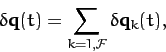(800)

where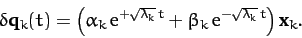(801)

Here, the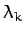and the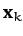are the eigenvalues and eigenvectors obtained by solving Equation (786). Moreover, the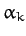and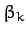are arbitrary constants. Finally, we have made use of the fact that the two roots of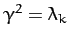are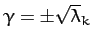.

According to Equation (800), the most general perturbed motion of the system consists of a linear combination of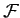different modes. These modes are generally termed normal modes, since they are mutually orthogonal (because theare mutually orthogonal). Furthermore, it follows from the independence of thethat the normal modes are also independent (i.e., one mode cannot be expressed as a linear combination of the others). The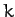th normal mode has a specific pattern of motion which is specified by theth eigenvector,. Moreover, theth mode has a specific time variation which is determined by the associated eigenvalue,. Recall thatis real. Hence, there are only two possibilities. Eitheris positive, in which case we can write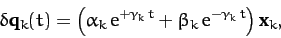(802)

where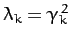, oris negative, in which case we can write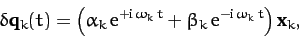(803)

where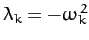. In other words, ifis positive then theth normal mode grows or decays secularly in time, whereas ifis negative then theth normal mode oscillates in time. Obviously, if the system possesses one or more normal modes which grow secularly in time then the equilibrium about which we originally expanded the equations of motion must be an unstable equilibrium. On the other hand, if all of the normal modes oscillate in time then the equilibrium is stable. Thus, we conclude that whilst Equation (773) is the condition for the existence of an equilibrium state in a many degree of freedom system, the condition for the equilibrium to be stable is that all of the eigenvalues of the stability equation (786) must be negative.

The arbitrary constantsandappearing in expression (801) are determined from the initial conditions. Thus, if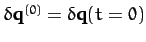and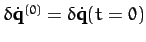then it is easily demonstrated from Equations (799)-(801) that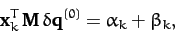(804)

and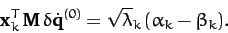(805)

Hence,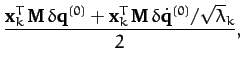(806)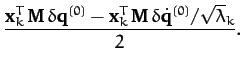(807)

Note, finally, that since there are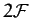arbitrary constants (two for each of thenormal modes), we can be sure that Equation (800) represents the most general solution to Equation (782).Next: Normal Coordinates Up: Coupled Oscillations Previous: More Matrix Eigenvalue Theory
Richard Fitzpatrick 2011-03-31